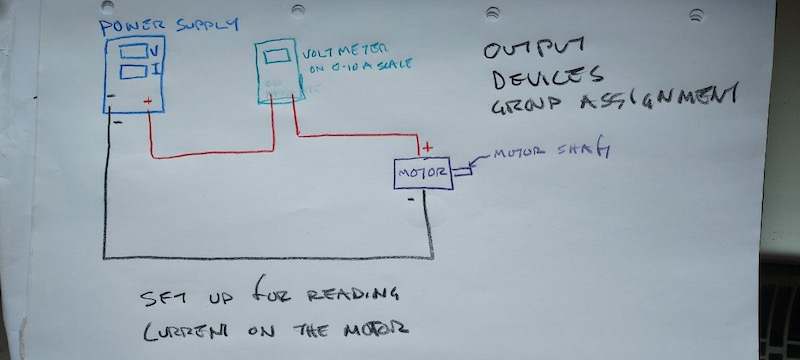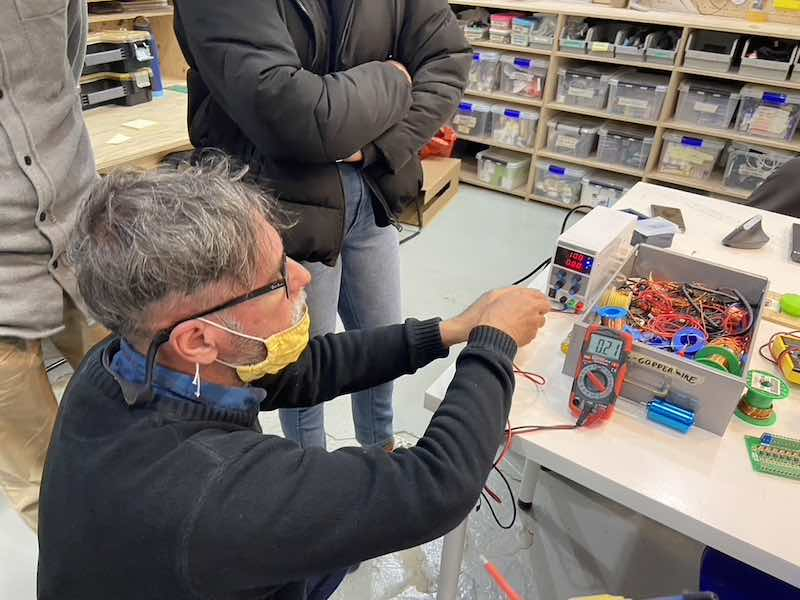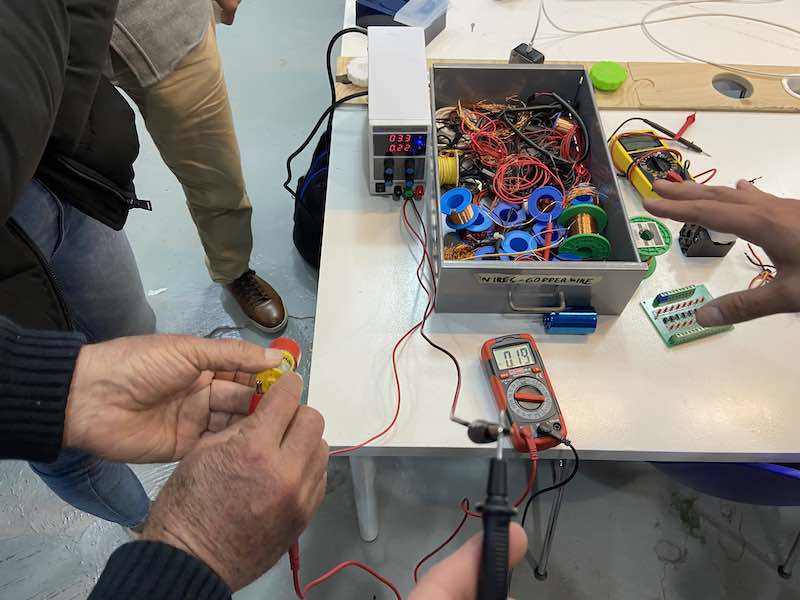# Output Devices

• group assignment:
• measure the power consumption of an output device

## Measuring the consumption of a Turbofan

This week we explored a variety of output devices and we use a multimeter to measure current consumption of a DC motor installed inside of a 3D printer turbofan that we found around the lab.

We also read the voltage and current of a small electric motor as we varied the torque on the motor by gripping the motor shaft while it was running. That way you can actually feel the increased current as increased twisting force on your fingers.

We used a power supply with adjustable voltage and maximum current settings to supply power to the motor. Meters on the power supply read voltage and current used by the motor.

Just for fun we also used a digital voltmeter to read the current. To read current, you need to break the circuit and put the moter in series with the voltmeter. Power is simply voltage multiplied by current.The top number on the power supply is the voltage (3.3 v in this case) and the bottom number is the current, which varies in each photo below as we varied the torque produced by the motor by gripping the shaft of the motor. Power is simply the voltage multiplied by the current.

Here’s how the current changed as we tightened the grip on the rotating shaft:

0.19 amps 3.3 volts 0.63 watts0.21 amps 3.3 volts 0.69 watts0.37 3.3 volts 1.221 wattsThere was a small discrepancy between the voltmeter amp reading the power supply amp reading. Likely the power supply is more accurate.